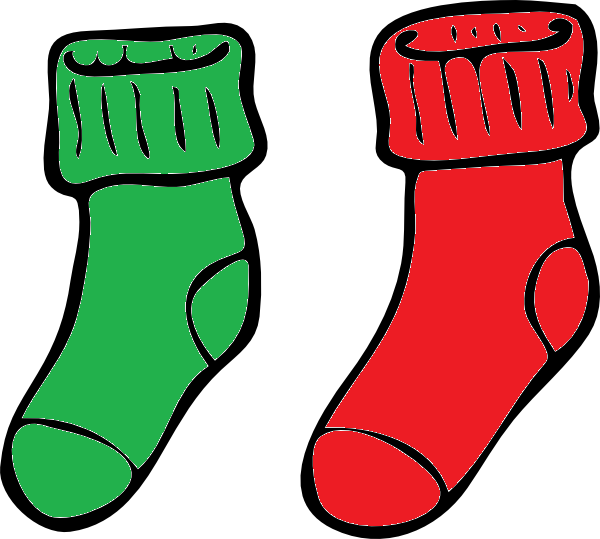# Solution: Odd Socks Puzzle

## The Puzzle:Sam is a bit of a slob when it comes to folding his laundry. He shoves all his socks, unfolded, into his drawer. He has eight socks in his drawer, some of which are red and some of which are green. If he reaches into his drawer without looking and takes out two socks, the probability of choosing a pair of green socks is $\dfrac{3}{4}$. What is the probability of choosing a pair of red socks?

## The Solution:

It's very tempting to say that the probability is $1 - \dfrac{3}{4} = \dfrac{1}{4}$, but this is incorrect. Don't forget that it is possible to remove two mismatched socks from the drawer. In fact, there must only be one red sock in the drawer, so the probability of choosing a red pair of socks is zero (there are no pairs of red socks to choose). Let's think about why.

Suppose, for a minute, that there are two red socks in the drawer. Then there must be $8 - 2 = 6$ green socks in the drawer. So, there is a $\dfrac{6}{8} = \dfrac{3}{4}$ chance of the first sock removed being green, and a $\dfrac{5}{7}$ chance of the second sock removed being green. Therefore, the chance of removing a pair of green socks is \(\dfrac{3}{4}\times \dfrac{5}{7} = \dfrac{15}{28}

### Description

This series of puzzles are for Year 10 or higher students, these puzzles tests your skills and also train you with problem solving and thinking out of the box

### Audience

Year 10 students or higher

### Learning Objectives

Solving puzzles

Author: Subject Coach
You must be logged in as Student to ask a Question.